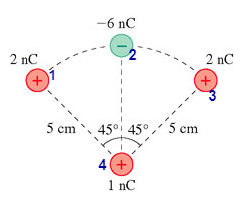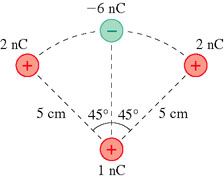# Problem: What is the force F on the 1 nC charge at the bottom? (Figure 1) Write your answer as two vector components, separated by a comma. Express each component numerically, in newtons, to three significant figures.

###### FREE Expert Solution

In this problem, we're going to use Coulomb's law to determine the force acting on 1 nC charge at the bottom.

Coulomb's law:

$\overline{){\mathbf{F}}{\mathbf{=}}\frac{\mathbf{k}{\mathbf{q}}_{\mathbf{1}}{\mathbf{q}}_{\mathbf{2}}}{{\mathbf{r}}^{\mathbf{2}}}}$, where F is the force between two charges q1 and q2, r is the distance between the two charges, and k is Coulomb's constant.

For easy working, let's label our diagram as follows:81% (450 ratings)###### Problem Details

What is the force F on the 1 nC charge at the bottom? (Figure 1) Write your answer as two vector components, separated by a comma. Express each component numerically, in newtons, to three significant figures.Frequently Asked Questions

What scientific concept do you need to know in order to solve this problem?

Our tutors have indicated that to solve this problem you will need to apply the Coulomb's Law (Electric Force) concept. You can view video lessons to learn Coulomb's Law (Electric Force). Or if you need more Coulomb's Law (Electric Force) practice, you can also practice Coulomb's Law (Electric Force) practice problems.